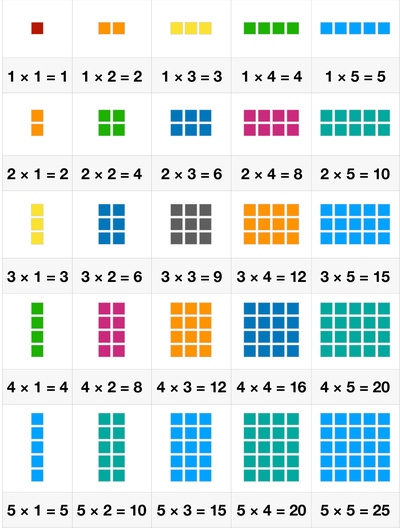# Number Blocks: Multiplications 5 by 5Addition, subtraction, multiplication and division are the four elementary mathematical operations of arithmetic. Multiplication is taking groups of a number together to get the total amount of those values combined. Essentially, multiplication is repeated addition. Usually kids start learning multiplication in the second or third grade. By then, they should have mastered the skills of counting and addition. Learning multiplication is extremely important, because it builds the foundation to understand division, fraction, and be able to spot patterns.

This worksheet illustrates multiplications of two small factors from 1 to 5. Each multiplication equation is illustrated with groups of blocks. It is a great tool to help kids understand the basic idea of multiplication: adding equal groups together. It is better to introduce multiplication to kids as soon as they start learning numbers and counting. With the help of this sheet, parents could help kids become familiar and confident with multiplications of small factors.